# What Is Rotational Symmetry?

Katherine Kaylegian-Starkey, David Karsner, Amanda Robb
• Author
Katherine Kaylegian-Starkey

Katherine has a bachelor's degree in physics, and she is pursuing a master's degree in applied physics. She currently teaches struggling STEM students at Lane Community College.

• Instructor
David Karsner

David holds a Master of Arts in Education

• Expert Contributor
Amanda Robb

Amanda has taught high school science for over 10 years. She has a Master's Degree in Cellular and Molecular Physiology from Tufts Medical School and a Master's of Teaching from Simmons College. She is also certified in secondary special education, biology, and physics in Massachusetts.

Learn the definition of rotational symmetry. Explore the rotational symmetry of different shapes, and understand what the angle and degree of rotation are. Updated: 02/21/2022

Show

## Rotational Symmetry

Imagine spinning a basketball like the player in Figure 1. No matter when the spin is stopped, the ball has the same shape. Now imagine rolling dice like in Figure 2. It doesn't matter which face is pointing toward the sky when the dice stop rolling, they will always look the same. These are examples of rotational symmetry.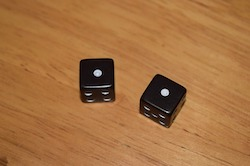## What is Rotational Symmetry?

The recycling icon is a very common symbol, and like most effective icons, the image itself is suggestive of its meaning. The arrows of the image appear to be moving in a circular manner, suggesting the circular concept of recycling. Adding to this perception is that if you were to rotate the image 120 degrees, and another 120 degrees, and a third 120 degrees, it would look the same at all three stops.

This attribute is called rotational symmetry. Many shapes have rotational symmetry, such as rectangles, squares, circles, and all regular polygons. Choose an object and rotate it up to 180 degrees around its center. If at any point the object appears exactly like it did before the rotation, then the object has rotational symmetry. In this lesson, you will be given several image examples, as well as definitions of the relevant concepts center, order, and degree of rotation.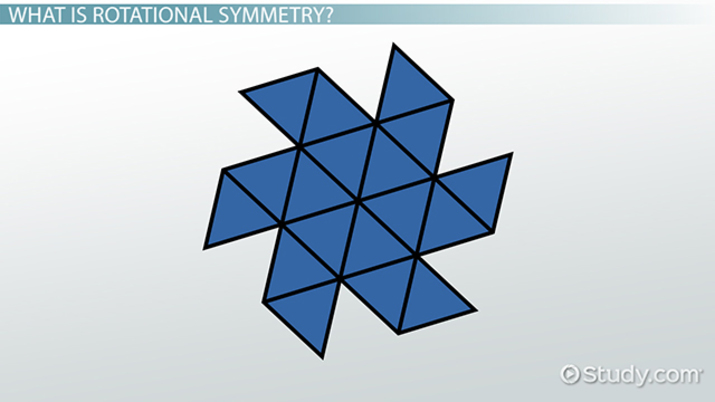An error occurred trying to load this video.

Try refreshing the page, or contact customer support.

Coming up next: Graphing Basic Functions

### You're on a roll. Keep up the good work!

Replay
Your next lesson will play in 10 seconds
• 0:01 What is Rotational Symmetry?
• 0:57 What is the Center of…
• 1:16 What is Order of Symmetry?
• 2:03 What is Degree of Rotation?
• 3:07 Lesson Summary
Save Save

Want to watch this again later?

Timeline
Autoplay
Autoplay
Speed Speed

## What Is Rotational Symmetry?

Rotational Symmetry Definition
As Figure 1 and Figure 2 illustrate, rotational symmetry involves congruity of a shape during a rotation. Also, for a shape to have rotational symmetry, the shape must look the same before and after a rotation of less than {eq}360^{\circ} {/eq}.
Determining What Rotational Symmetry Is
To know if a shape has rotational symmetry, answer the following questions—if the answer to each bullet point is yes, then the shape in question has rotational symmetry:
• Is the angle of rotation, explained in the following section, less than {eq}360^{\circ} {/eq}?
• If the angle of rotation is less than {eq}360^{\circ} {/eq}, does the after-rotation shape look identical to the before-rotation shape?

### Angle of Rotational Symmetry

The angle of rotational symmetry is how much a shape must be rotated before it looks the same as it did before the rotation. Return to the basketball example of the introduction. Mark a spot on the basketball, and mark a line on the floor. Align the spot and the line, then spin the basketball and mark another line where the spot on the basketball comes to rest. The angle that is formed between these two lines is the angle of rotational symmetry.

The basketball example illustrates continuous rotational symmetry because, no matter how big or how small the angle of rotation is, the basketball will always look the same. The dice of Figure 2, on the other hand, must roll in multiples of {eq}90^{\circ} {/eq} to have rotational symmetry because, only if the dice roll from one face to another, is there symmetry. This is an example of non-continuous rotational symmetry.

### Degree of Rotational Symmetry

The degree of rotational symmetry, also called the order of rotational symmetry, refers to how many times in {eq}360^{\circ} {/eq} a shape can be rotated and display rotational symmetry. For a regular polygon, the order of rotational symmetry is equal to the number of sides of the polygon. Refer again to the basketball whose symmetry is continuous. This sphere can be rotated infinitesimally and always look the same, and all spheres have infinite rotational symmetry. Now think about the dice again. The dice that must be rotated in multiples of {eq}90^{\circ} {/eq} can only be rotated four times before completing a circle. These cubes, like all cubes, have rotational symmetry of order 4.

Another measure of rotational symmetry looks at how many different axes a shape can be rotated around and exhibit rotational symmetry. A basketball has only a single axis, and therefore it can only rotate about that single axis. The dice have six sides, eight vertices, and thirteen possible axes of rotation.

### Rotational Symmetry Shapes

Many shapes have rotational symmetry of at least order 1 with at least one axis of rotation. Figure 3, Figure 4, and Figure 5 illustrate different rotational symmetry shapes. Figure 3 is a tetrahedron which has 24 axes of rotation and a rotational order of 4.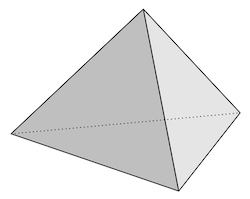Figure 4 is a 5-sided star, and, because of its five sides, the star has a rotational symmetry of order 5.Figure 5 is a rhombus. This shape does not follow the regular polygon rule, and a rhombus has a rotational symmetry order of 2 and 2 axes of rotation.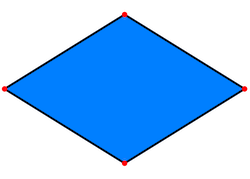### Rotational Symmetry Graph

Some functions, just like some geometric shapes, exhibit rotational symmetry, and identifying functions that have this symmetry can greatly simplify complex calculus problems. For example, any odd function has rotational symmetry about the origin. Recall that, for an odd function, {eq}f(-x) = -f(x) {/eq}. Figure 6 is the cubic function {eq}f(x) = 5x^3 {/eq}. This function is odd, and, when it is rotated {eq}180^{\circ} {/eq} about the origin, this function exhibits rotational symmetry.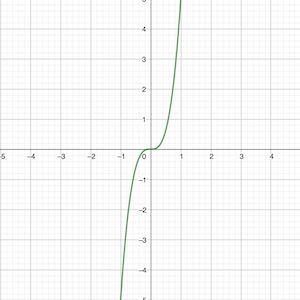Figure 7 is another rotational symmetry graph. This is the plot of {eq}sin(x) {/eq}, and the rotational symmetry of this function is commonly used by physicists to simplify wave function calculations.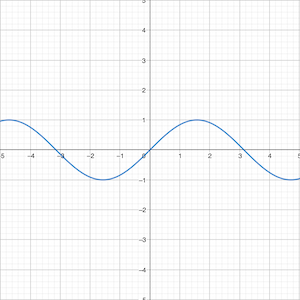## How to Find Rotational Symmetry

When analyzing a geometric shape, it is useful to know the angle of rotational symmetry and the degree, or order, of rotational symmetry. To find these attributes, use the following set of steps for how to find rotational symmetry:

1. Identify if the shape has symmetry before and after rotation. If it does, ensure that the shape has not been rotated 360°.
2. Determine the angle of rotation. This can be accomplished by identifying the pivot point of the shape then using trigonometry.
3. Once the angle of rotation is known, divide 360° by the angle of rotation. The result is the order of rotational symmetry. For example, for a cube with a 90° angle of rotation, {eq}\frac{360^{\circ}}{90^{\circ}} = 4 {/eq} which is the correct order of rotational symmetry for a cube.

## What is the Center of an Object?

The center of a shape or object with rotational symmetry is the point around which the rotation occurs. If one was to spin a basketball on the tip of his finger, the tip of his finger would be the center of the rotational symmetry. If an object has rotational symmetry, its center will also be its center of balance.

## Order of Symmetry

The order of symmetry - or for short, order - is the number of times an object or shape can be rotated and still look like it did before rotation began. Let's look at some examples.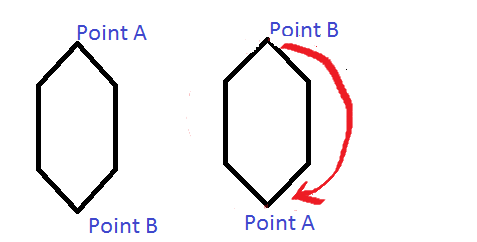Here we have a hexagon. It has an order of 2. Let's look at another example.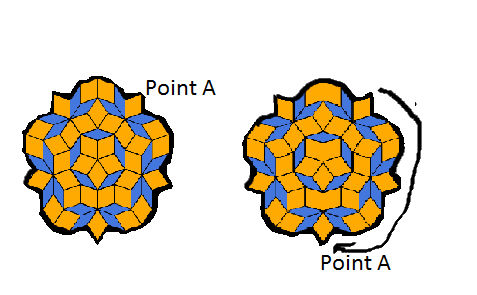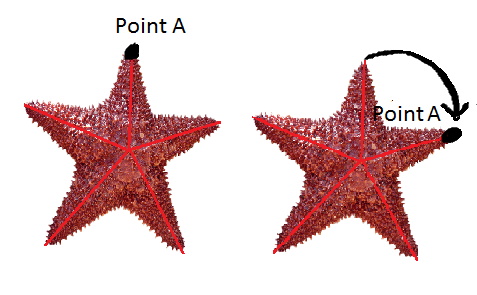Both the blue and orange shape and starfish have an order of 5 because you can turn them 5 times and they still look the same as they did before they were rotated.

Let's look at one more example.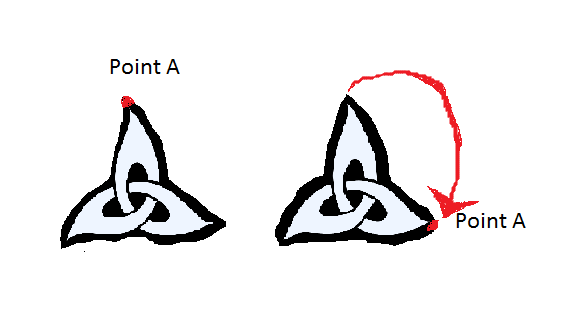This one has an order of 3.

The smallest order would be an order of 2. You cannot have a shape or object that has an order of 1. An order of 1 would mean that you can complete a full rotation without it appearing as it did before the rotation. In other words, an order of 1 would mean that is has no rotational symmetry.

To unlock this lesson you must be a Study.com Member.

Video Transcript

## What is Rotational Symmetry?

The recycling icon is a very common symbol, and like most effective icons, the image itself is suggestive of its meaning. The arrows of the image appear to be moving in a circular manner, suggesting the circular concept of recycling. Adding to this perception is that if you were to rotate the image 120 degrees, and another 120 degrees, and a third 120 degrees, it would look the same at all three stops.

This attribute is called rotational symmetry. Many shapes have rotational symmetry, such as rectangles, squares, circles, and all regular polygons. Choose an object and rotate it up to 180 degrees around its center. If at any point the object appears exactly like it did before the rotation, then the object has rotational symmetry. In this lesson, you will be given several image examples, as well as definitions of the relevant concepts center, order, and degree of rotation.

## What is the Center of an Object?

The center of a shape or object with rotational symmetry is the point around which the rotation occurs. If one was to spin a basketball on the tip of his finger, the tip of his finger would be the center of the rotational symmetry. If an object has rotational symmetry, its center will also be its center of balance.

## Order of Symmetry

The order of symmetry - or for short, order - is the number of times an object or shape can be rotated and still look like it did before rotation began. Let's look at some examples.Here we have a hexagon. It has an order of 2. Let's look at another example.Both the blue and orange shape and starfish have an order of 5 because you can turn them 5 times and they still look the same as they did before they were rotated.

Let's look at one more example.This one has an order of 3.

The smallest order would be an order of 2. You cannot have a shape or object that has an order of 1. An order of 1 would mean that you can complete a full rotation without it appearing as it did before the rotation. In other words, an order of 1 would mean that is has no rotational symmetry.

To unlock this lesson you must be a Study.com Member.

• Activities
• FAQs

## Rotational Symmetry and Art

In this creative project, students will create a work of art that has rotational symmetry. Students can use any medium they desire, such as clay, paint, colored pencils, or collage. However, the art that students create should have rotational symmetry and be able to be hung in any direction, as described in the lesson. For example, students might create a mandala like shape from cut outs of colored paper in a color scheme they enjoy. This would be an example of rotational symmetry.

### Student Instructions

Now that you understand what rotational symmetry is and how to identify it, it's time to try your own hand at creating something with this type of symmetry. In this creative assignment you will be creating a work of art that has rotational symmetry. You can choose any medium to work with, such as paint, clay, collage, or something else of your choosing. However, your end product should have rotational symmetry. For a list of constraints, see the criteria for success below.

#### Criteria For Success

• Art should have rotational symmetry
• Art should include a color scheme that is attractive
• The student should be able to discuss their vision for the piece and explain why it has rotational symmetry

#### How do you find the rotational symmetry of a shape?

Rotational symmetry means that a shape or a function can be rotated about a point and look the same as it did before the rotation. To find the rotational symmetry of a shape, first identify if the shape looks the same as it did before the rotation. If it does, make sure the shape was turned less than 360°. If it has been, the shape has rotational symmetry.

#### What is rotational symmetry Order 3?

Rotational symmetry order 3 means that a shape can be rotated 3 times within a full circle and look the same as it did before the rotation. For a shape to be of order 3, it must have an angle of rotation of 120°.

#### What is the rotational symmetry in math?

Rotational symmetry in math means that a shape can be spun around a single point and look the same as it did before it was spun. Many shapes and functions exhibit rotational symmetry.

#### How do you explain rotational symmetry?

Rotational symmetry means that a shape or a function can be spun about a point and look the same as it did before it was spun. Regular polygons and odd functions exhibit rotational symmetry.

### Register to view this lesson

Are you a student or a teacher?

Back

### Resources created by teachers for teachers

Over 30,000 video lessons & teaching resources‐all in one place.Video lessonsQuizzes & WorksheetsClassroom IntegrationLesson Plans

I would definitely recommend Study.com to my colleagues. It’s like a teacher waved a magic wand and did the work for me. I feel like it’s a lifeline.

Jennifer B.
TeacherCreate an account to start this course today
Used by over 30 million students worldwide